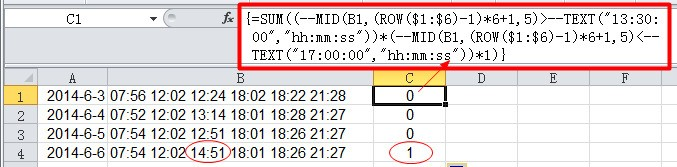• ZOL首页
• 产品报价
• 论坛
• ZOL问答
• 更多
• 手机客户端

• 回答数

5

• 浏览数

10,679

### 5个回答 默认排序 默认排序 按时间排序

=COUNTIF(A:A,A1)>1

=countif(a:a,a1)……然后 自动筛选 筛选这个辅助列 >=5……你也可以再变通一下 公式结果 可以用=countif(a:a,a1)>=5 然后筛选 true……

E2 =countif(a:a,a2)>=5 然后 双击E2 单元格 右下角的小黑十字 向下复制填充 或者 下拉复制……

C列大于0的就是包含有这个时间段的行。
=SUM((--MID(B1,(ROW(\$1:\$6)-1)*6+1,5)>--TEXT("13:30:00","hh:mm:ss"))*(--MID(B1,(ROW(\$1:\$6)-1)*6+1,5)<--TEXT("17:00:00","hh:mm:ss"))*1)B1单元格写入公式
=IF(AND(COUNTIF(A\$1:A1,A1)=1,COUNTIF(A:A,A1)>4),"是","")

C1单元格写入公式
=INDEX(A:A,SMALL(IF(B\$1:B\$1000="是",ROW(\$1:\$1000),4^8),ROW(A1)))&""

C列的内容就是你要的内容了

B,C列如果有内容,你可以在A列后插入两个新列
=countif（A：A，A1）大于1的有重复

### 最新回答 更多>

ZOL问答 > 在Excel表格中有两千多个名字,怎么快速筛选出重名的人,而且要知道具体在哪行重名?

### 举报成功